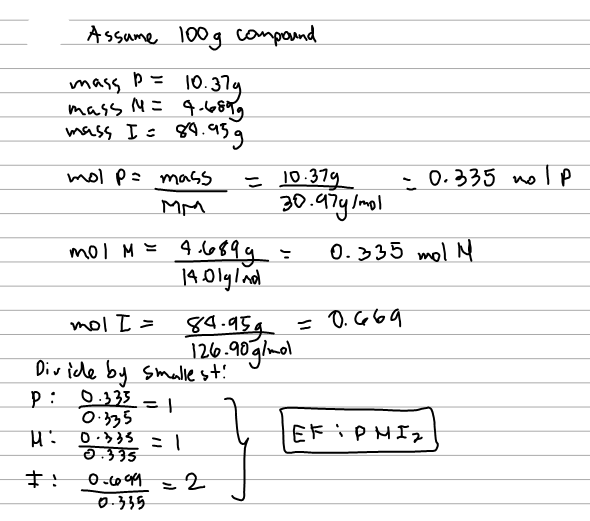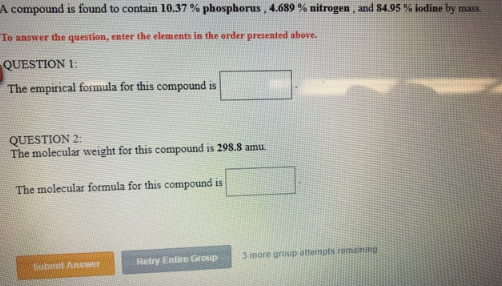# Problem: A compound is found to contain 10.37% phosphorus, 4.689% nitrogen , and 84.95% iodine by mass. To answer the question, enter the elements in the order presented above. QUESTION 1:The empirical formula for this compound is ___________.QUESTION 2:The molecular weight for this compound is 298.8 amu. The molecular formula for this compound is ___________.

###### FREE Expert Solution100% (37 ratings)###### Problem Details

A compound is found to contain 10.37% phosphorus, 4.689% nitrogen , and 84.95% iodine by mass.

To answer the question, enter the elements in the order presented above.

QUESTION 1:

The empirical formula for this compound is ___________.

QUESTION 2:

The molecular weight for this compound is 298.8 amu.

The molecular formula for this compound is ___________.